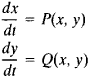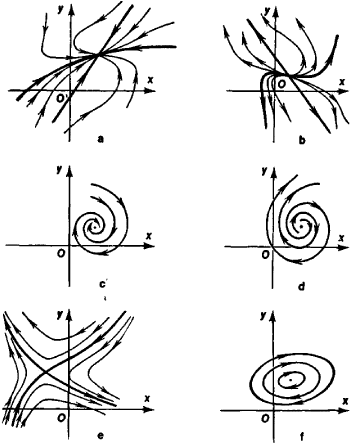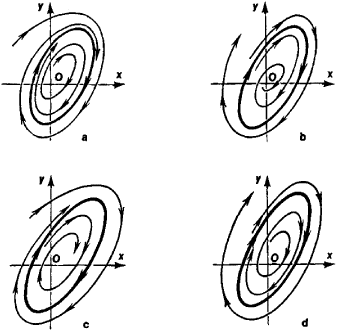# Phase Plane Analysis

## phase plane analysis

[′fāz ¦plān ə′nal·ə·səs]
(control systems)
A method of analyzing systems in which one plots the time derivative of the system's position (or some other quantity characterizing the system) as a function of position for various values of initial conditions.
McGraw-Hill Dictionary of Scientific & Technical Terms, 6E, Copyright © 2003 by The McGraw-Hill Companies, Inc.
The following article is from The Great Soviet Encyclopedia (1979). It might be outdated or ideologically biased.

## Phase Plane Analysis

a graphical method of analyzing dynamic systems described by equations of the typewhere x and y are the state variables of the system, P(x, y) and Q(x, y) are functions that satisfy the conditions for the existence and uniqueness of solutions, and t is time—the independent variable. The behavior of such a system may be represented geometrically in a plane in rectangular Cartesian coordinates. In such a representation, a point in the xy-plane uniquely corresponds to each state of the dynamic system; conversely, one and only one state of the dynamic system being analyzed corresponds to each point in the plane. The plane Oxy is called the phase plane. AFigure 1. Phase trajectories in the neighborhood of the following types of singular points: (a) a stable node, (b) an unstable node, (c) a stable focus, (d) an unstable focus, (e) a saddle point, (f) a center

change in the state of the system is represented in the phase plane by the motion of a point, which is called the phase point, or the point of representation or presentation. The trajectory along which the phase point moves is called a phase trajectory. The rate and direction of the phase point’s motion are given by the phase velocity vector {P, Q}. It is significant that only one phase trajectory passes through each point of the phase plane. A set of phase trajectories is called a phase portrait of a system and represents the set of all possible configurations of the system and the types of possible motions in the system.

The following three types of phase trajectories are usually distinguished in a phase plane: singular points, or equilibrium positions, which are determined by solving the system of equations P(x, y) = 0, Q(x, y) = 0; isolated closed trajectories, which correspond to periodic motions in the system; and separatrices, which divide the phase plane into regions occupied by different types of trajectories.

Phase plane analysis consists in the construction of the phase portrait of the system and the subsequent analysis of the phase portrait. The technique makes it possible to determine the number,Figure 2. Phase trajectories in the neighborhood of various limit cycles, which are depicted as closed curves: (a) a stable limit cycle, (b) an unstable limit cycle, (c) and (d) semistable limit cycles

types, and nature of singular points, isolated closed trajectories, and separatrices and to represent graphically, on the basis of the shape of the phase trajectories, the complete set of motions that occur in a dynamic system under all possible initial conditions. Singular points are classified according to the nature of the phase trajectories in their neighborhood. The main types of singular points are illustrated in Figure 1. Isolated closed trajectories, or limit cycles, are classified according to the nature of their stability (Figure 2).

In combination with analytical methods, phase plane analysis makes it possible to obtain quantitative estimates of the solutions of the differential equations that describe a dynamic system. For example, phase plane analysis may be used to estimate the time required for a phase point to undergo a transition from one state to another—that is, to estimate the duration of a transition process—or to determine the period and “amplitude” of a periodic motion.

The theoretical principles of phase plane analysis were developed by H. Poincaré. Phase plane analysis is a technique of the qualitative theory of dynamic systems. It is widely used in the theory of oscillations, automatic control theory, electrical engineering, and mechanics.

### REFERENCES

Poincaré, H. O krivykh, opredeliaemykh differentsial’nymi uravneniiami. Moscow-Leningrad, 1947. (Translated from French.)
Nemytskii, V. V., and V. V. Stepanov. Kachestvennaia teoriia differentsial’nykh uravnenii, 2nd ed. Moscow-Leningrad, 1949.
Andronov, A. A., A. A. Vitt, and S. E. Khaikin. Teoriia kolebanii, 2nd ed. Moscow, 1959.
Kachestvennaia teoriia dinamicheskikh sistem vtorogo poriadka, 2nd ed. Moscow, 1966.
Emel’ianov, S. V. Sistemy avtomaticheskogo upravleniia s peremennoi strukturoi. Moscow, 1967.
Marchukov, B. A. Proektirovanie sistem upravleniia metodami fazovoi ploskosti. Moscow, 1976.

S. K. KOROVIN and N. N. MILOVIDOV